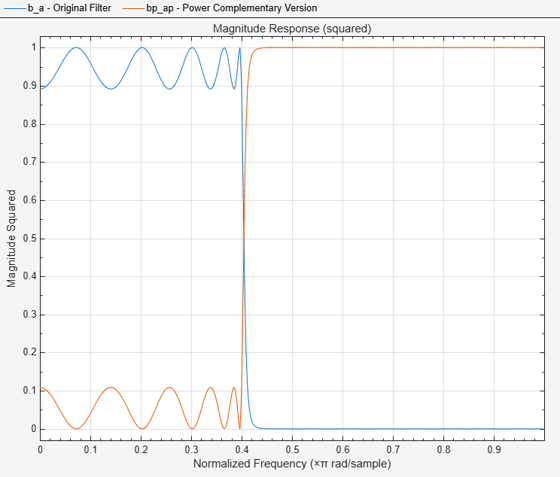iirpowcomp

Power complementary IIR filter

Description

example

[bp,ap] = iirpowcomp(b,a) returns the coefficients vectors bp and ap, of the power complementary IIR filter g(z) = bp(z) / ap(z), given the coefficients vectors b and a of the IIR filter h(z) = b(z)/ a(z) in . The vector b must be symmetric (Hermitian) or antisymmetric (antihermitian) and of the same length as the vector a. The two power complementary filters satisfy the relation

|H(w)|2 + |G(w)|2 = 1.

[bp,ap] = iirpowcomp(b,a,c) allow you to specify a complex scalar of unity magnitude, c, which forces bp to satisfy the generalized Hermitian property:

conj(bp(end:-1:1)) = c*bp

Examples

collapse all

Calaculate the coefficients of the power complementary IIR filter.

[b,a]=cheby1(10,.5,.4);
[bp,ap]=iirpowcomp(b,a);

Compare the magnitude responses of the filters using FVTool.

fvtool(b,a,bp,ap,'MagnitudeDisplay','Magnitude squared');
legend('Original Filter','Power Complementary Version');Input Arguments

collapse all

Numerator coefficients of the IIR filter, specified as a row vector. The vector b must be symmetric (Hermitian) or antisymmetric (antihermitian) and of the same length as the vector a.

Data Types: single | double
Complex Number Support: Yes

Denominator coefficients of the IIR filter, specified as a row vector.

Data Types: single | double
Complex Number Support: Yes

Complex scalar of unity magnitude, which forces the bp to satisfy generalized Hermitian property.

The generalized Hermitian property is given by:

conj(bp(end:-1:1)) = c*bp

When c is omitted, the function chooses c as follows:

• When b is real, the function chooses c as 1 or -1, whichever yields bp as real.

• When b is complex, c defaults to 1.

ap is always equal to a.

Data Types: single | double
Complex Number Support: Yes

Output Arguments

collapse all

Numerator coefficients of the power complementary IIR filter, returned as a row vector.

Data Types: single | double

Denominator coefficients of the power complementary IIR filter, returned as a row vector.

Data Types: single | double
Complex Number Support: Yes